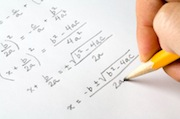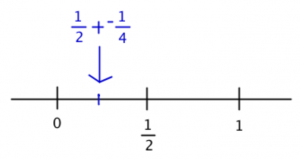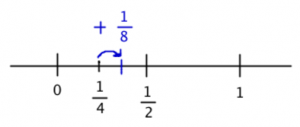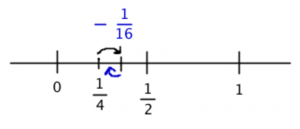# Want a 51 on Quant? Answer This Problem!

by , Jan 24, 2014Sequence problems arent incredibly common on the test, but if youre doing well on the quant section, be prepared to see one. Now, youve got a choice: do you want to guess quickly and save time for other, easier topics? Do you want to learn some test savvy techniques that will help you with some sequence questions but possibly not all of them? Or do you want to learn how to do these every single time, no matter what?

That isnt a trick question. Every good business person knows that theres a point of diminishing returns: if you dont actually need a 51, then you may study for a lower (but still good!) score and re-allocate your valuable time elsewhere.

Try this GMATPrep problem from the free test. After, well talk about how to do it in the textbook way and in the back of the envelope way.

* For every integer k from 1 to 10, inclusive, the kth term of a certain sequence is given by [pmath](-1)^{k+1}(1/2^k)[/pmath]. If T is the sum of the first 10 terms in the sequence, then T is

(A) greater than 2

(B) between 1 and 2

(C) between [pmath]1/2[/pmath] and 1

(D) between [pmath]1/4[/pmath]and[pmath]1/2[/pmath]

(E) less than [pmath]1/4[/pmath]

First, lets talk about how to do this thing in the textbook math way. If you dont want to do this the textbook math way, feel free to skip to the second method below.

## Textbook Method

If youve really studied sequences, then you may recognize the sequence as a particular kind called a Geometric Progression. If not, you would start to find the terms and see whether you can spot a pattern.

Plug in k = 1, 2, 3. Whats going on?

If k = 1, then[pmath](-1)^{1+1}(1/2^1)=(1)(1/2)=1/2[/pmath]

If k = 2, then[pmath](-1)^{2+1}(1/2^2)=(-1)(1/4)=-1/4[/pmath]

If k = 3, then[pmath](-1)^{3+1}(1/2^3)=(1)(1/8)=1/8[/pmath]

Whats going on here? Each time, the term gets multiplied by [pmath]-1/2[/pmath]in order to get to the next one. When you keep multiplying by the same number in order to get to the next term, then you have a geometric progression.

This next part gets into some serious math. Unless you really just love math, I wouldnt bother learning this part for the GMAT, because theres a very good chance youll never need to use it. But, if you want to, go for it!

When you have a geometric progression, you can calculate the sum in the following way:

[pmath]s=1/2+(-1/4)+1/8+(-1/16)+...[/pmath]

Next, youre going to multiply every term in the sum by the common ratio. Whats the common ratio? Its the constant number that you keep multiplying each term by to get the next one. In this case, youve already figured this out: its [pmath](-1/2)[/pmath].

If you multiply this through all of the terms on both sides of the equation, youll get this:

[pmath](-1/2)s=(-1/4)+1/8+(-1/16)+...[/pmath]

Does anything look familiar? Its basically the same list of numbers as in the first sum equation, except its missing the first number, [pmath]1/2[/pmath]. All of the others are identical!

Subtract this second equation from the first:

[pmath]s-(-1/2)s=1/2[/pmath]

The right-hand side of the equation is always going to be just the first term of the original sum. The rest of the terms on the right-hand side of the two equations are identical, so when you subtract, they become zero and disappear.

Solve for s:

[pmath]{3/2}s=1/2[/pmath]

[pmath]s=1/3[/pmath]

This value falls between 1/4 and 1/2, so the answer is (D).

## Back of the Envelope Method

There is another way to tackle this one. At the same time, this problem is really trickyso this solution is still not an easy solution. Your best choice might be just to guess and move on.

Before you start reading the text, take a First Glance at the whole thing. Its a problem-solving problem. The answers are weird. Theyre not exact. What does that mean?

Read the problem, but keep that answer weirdness in mind. The first sentence has a crazy sequence. The question asks you to sum up the first 10 terms of this sequence. And the answers arent exact so apparently you dont need to find the exact sum.

Take a closer look at the form of the answers. Notice anything about them?

They dont overlap! They cover adjacent ranges. If you can figure out that, for example, the sum is about 3/4, then you know the answer must be (C). In other words, you can actually estimate hereyou dont have to do an exact calculation.

That completely changes the way you can approach this problem! Heres the sequence:

[pmath](-1)^{k+1}(1/2^k)[/pmath]

According to the problem, the 10 terms are from k = 1 to k = 10. Calculating all 10 of those and then adding them up is way too much work (another clue that theres got to be a better way to do this one). So what is that better way?

Since you know you can estimate, try to find a pattern. Calculate the first two terms (we had to do this in the first solution, too).

Ifk= 1, then[pmath](-1)^{1+1}(1/2^1)=(1)(1/2)=1/2[/pmath]

Ifk= 2, then[pmath](-1)^{2+1}(1/2^2)=(-1)(1/4)=-1/4[/pmath]

Whats going on? The first answer is positive and the second one is negative. Why? Ah, because the first part of the calculation is -1 raised to a power. That will just keep switching back and forth between 1 and -1, depending on whether the power is odd or even. It wont change the size of the final answer, but it will change the sign.

Okay, and what about that second part? it went from 1/2 to 1/4. What will happen next time? Try just that part of the calculation. If k = 3, then just that part will become [pmath](1/2^3)=1/8[/pmath].

Interesting! So the denominator will keep increasing by a factor of 2: 2, 4, 8, 16 and so on.

Great, now you can write out the 10 numbers!

[pmath]1/2,{-1/4},1/8,{-1/16},1/32,{-1/64}[/pmath] ugh. The denominators getting pretty big. That means the fraction itself is getting pretty small. Do I need to keep writing these out?

What was the problem asking again?

Right, find the sum of these 10 numbers. Lets see. The first number in the sequence is 1/2 and the second is -1/4, so the pair adds up to 1/4.Right now, the answer would be right between D and E. Does the sum go up or down from here?

The third number will add 1/8, so it goes up:But the fourth will subtract 1/16 (dont forget that every other term is negative!), pulling it back down again:Hmm. In the third step, it went up but not enough to get all the way to 1/2. Then, it went down again, but by an even smaller amount, so it didnt get all the way back down to 1/4.

The fifth step would go up by an even smaller amount (1/32), and then it would go back down again by yet a smaller number (1/64). What can you conclude?

First, the sum is always growing a little bit, because each positive number is a bit bigger than the following negative number. The sum is never going to drop below 1/4, so cross off answer (E).

You keep adding smaller and smaller amounts, though, so if the first jump of 1/8 wasnt enough to get you up to 1/2, then none of the later, smaller jumps will get you there either, especially because you also keep subtracting small amounts. Youre never going to cross over to 1/2, so the sum has to be between 1/4 and 1/2.